# User:Tohline/Appendix/Ramblings/BordeauxSequences

(Difference between revisions)
Jump to: navigation, search
 Revision as of 23:01, 22 November 2020 (view source)Tohline (Talk | contribs) (→Surrounding Torus)← Older edit Revision as of 12:30, 23 November 2020 (view source)Tohline (Talk | contribs) (→Basillais & Huré (2019))Newer edit → Line 197: Line 197:
- $~4\pi G\biggl( \frac{2^3\pi}{3\cdot 5}\biggr)^2 (1 - e^2) R_\mathrm{eq}^{10} \rho^3 \Omega^2 \, .$ + $~4\pi G\biggl( \frac{2^3\pi}{3\cdot 5}\biggr)^2 (1 - e^2) R_\mathrm{eq}^{10} \rho^3 \Omega^2$ +
+   + + $~=$ + + $~\biggl( \frac{2^8 \pi^3}{3^2 \cdot 5^2}\biggr) (1 - e^2) G R_\mathrm{eq}^{10} \rho^3 \Omega^2 \, .$
- $~\frac{a}{R_\mathrm{eq}}$ + $~\alpha_t \equiv \frac{a}{R_\mathrm{eq}}$ Line 302: Line 314: $~2\pi a (\pi b^2)\rho [itex]~2\pi a (\pi b^2)\rho = = - 2\pi^2 R_\mathrm{eq}^3 \rho \biggl[ \frac{a}{R_\mathrm{eq}} \biggl( \frac{b}{R_\mathrm{eq}} \biggr)^2 \biggr] + 2\pi^2 R_\mathrm{eq}^3 \rho \biggl[ \alpha_t \beta_t^2 \biggr] - \, .$ + \, ,[/itex] +
+ + + + + + Line 278: Line 290: + + + where, + + +
+ $~\beta_t$ + + $~\equiv$ + + $~\frac{b}{R_\mathrm{eq}} \, .$
+ + + - Given that the major radius of the torus, $~a$, is known once the eccentricity of the central Maclaurin spheroid has been selected, and the density of the torus must match the density of the central object, the mass of the torus will only depend on the choice of $~b$.  The maximum allowed value of $~b$ is set by ensuring that the torus lies entirely outside of the central spheroid.  This means, + Given that $~\alpha_t$ is known once the eccentricity of the central Maclaurin spheroid has been selected and, given that the density of the torus must match the density of the central object, the mass of the torus will only depend on the choice of $~0 < \beta_t \le \beta_\mathrm{max}$.  The maximum allowed value, $~\beta_\mathrm{max}$, is set by ensuring that equatorial-plane location of the inner edge of the torus is no smaller than the equatorial radius of the central spheroid, $~R_\mathrm{eq}$.  This means,
- $~b_\mathrm{max}$ + $~\beta_\mathrm{max}$ Line 319: Line 346: - $~R_\mathrm{eq}\biggl[\frac{a}{R_\mathrm{eq}}-1 \biggr]$ + $~\alpha_t - 1 \, .$
- $~ + [itex]~ - 2\pi^2 R_\mathrm{eq}^3 \rho ~ + 2\pi^2 R_\mathrm{eq}^3 \rho \biggl[ \alpha_t \beta_\mathrm{max}^2 \biggr] - \biggl[\frac{1}{3\Omega^2} (1 - e^2)^{1 / 2} \biggr]^{1 / 3} \biggl\{ \biggl[\frac{1}{3\Omega^2} (1 - e^2)^{1 / 2} \biggr]^{1 / 3} -1 + = - \biggr\}^2 + 2\pi^2 R_\mathrm{eq}^3 \rho ~\alpha_t (\alpha_t - 1)^2 \, . - \, . +$ [/itex] $~M_t R_\mathrm{eq}^2\biggl[ \biggl(\frac{a}{R_\mathrm{eq}} \biggr)^2 + \frac{3}{4} \biggl( \frac{b}{R_\mathrm{eq}} \biggr)^2 \biggr]$ $~M_t R_\mathrm{eq}^2\biggl[ \biggl(\frac{a}{R_\mathrm{eq}} \biggr)^2 + \frac{3}{4} \biggl( \frac{b}{R_\mathrm{eq}} \biggr)^2 \biggr]$ +
+   + + $~=$ + + $~2\pi^2 R_\mathrm{eq}^5 \rho \alpha_t \beta_t^2 \biggl( \alpha_t^2 + \frac{3}{4} \beta_t^2 \biggr) \, .$ +
Line 334: Line 361: Line 356: Line 382: + + + + + + + + + Hence, the (square of the) angular momentum of the torus is, + + +
+ $~L_t^2 = I_t^2 \omega_0^2$ + + $~=$ + + $~ + \biggl[ 2\pi^2 R_\mathrm{eq}^5 \rho \alpha_t \beta_t^2 \biggl( \alpha_t^2 + \frac{3}{4} \beta_t^2 \biggr) \biggr]^2 \Omega^2 (4\pi G\rho) +$ +
+   + + $~=$ + + $~ + 2^4 \pi^5 \alpha_t^2 \beta_t^4 \biggl( \alpha_t^2 + \frac{3}{4} \beta_t^2 \biggr)^2 G R_\mathrm{eq}^{10} \rho^3 \Omega^2 \, . +$
+ $~\bar{a}^3$ + + $~=$ + + $~R_\mathrm{eq}^2 Z = R_\mathrm{eq}^3(1-e^2)^{1 / 2} \, ,$ +
+ + + + + + + + + Line 361: Line 430: =====Combined Configuration===== =====Combined Configuration===== - The total (maximum) mass of the system is, + + Given that, for the chosen Maclaurin spheroid, + + + + + + + + + + and that the total mass of the system is, Line 373: Line 459:
- $~M_c + M_\mathrm{max}$ + $~M_c + M_t$
$~ [itex]~ - \frac{4\pi}{3} \rho R_\mathrm{eq}^3 (1 - e^2)^{1 / 2} + \frac{2^2\pi}{3} \rho R_\mathrm{eq}^3 (1 - e^2)^{1 / 2} + + - 2\pi^2 R_\mathrm{eq}^3 \rho ~ + 2\pi^2 R_\mathrm{eq}^3 \rho \alpha_t \beta_t^2 - \biggl[\frac{1}{3\Omega^2} (1 - e^2)^{1 / 2} \biggr]^{1 / 3} \biggl\{ \biggl[\frac{1}{3\Omega^2} (1 - e^2)^{1 / 2} \biggr]^{1 / 3} -1 +$ - \biggr\}^2 \, . +
+   + + $~=$ + + $~ + 2\pi R_\mathrm{eq}^3 \rho \biggl[ \frac{2}{3} (1 - e^2)^{1 / 2} + \pi \alpha_t \beta_t^2 \biggr] + \, ,$ [/itex]
Line 386: Line 472: + + + + + + + the (square of the) dimensionless total angular momentum of the combined system is, + + +
+ $~j_\mathrm{tot}^2$ + + $~\equiv$ + + $~ + \biggl(\frac{3}{2^8 \pi^4}\biggr)^{1 / 3} \frac{(L_c + L_t)^2}{GM_\mathrm{tot}^3 \bar{a}} +$ +
+   + + $~=$ + + $~ + \biggl(\frac{3}{2^8 \pi^4}\biggr)^{1 / 3} + \biggl\{ L_c + L_t \biggr\}^2 \biggl\{ M_\mathrm{tot} \biggr\}^{-3}G^{-1} R_\mathrm{eq}^{-1} (1 - e^2)^{-1 / 6} +$ +
+   + + $~=$ + + $~ + \biggl(\frac{3}{2^8 \pi^4}\biggr)^{1 / 3} + \biggl\{ \biggl( \frac{2^8 \pi^3}{3^2 \cdot 5^2}\biggr)^{1 / 2} (1 - e^2)^{1 / 2} G^{1 / 2} R_\mathrm{eq}^{5} \rho^{3 / 2} \Omega + + 2^2 \pi^{5 / 2} \alpha_t \beta_t^2 \biggl( \alpha_t^2 + \frac{3}{4} \beta_t^2 \biggr) G^{1 / 2} R_\mathrm{eq}^{5} \rho^{3 / 2} \Omega \biggr\}^2 +$ +
+   + +   + + $~\times + \biggl\{ 2\pi R_\mathrm{eq}^3 \rho \biggl[ \frac{2}{3} (1 - e^2)^{1 / 2} + \pi \alpha_t \beta_t^2 \biggr] \biggr\}^{-3}G^{-1} R_\mathrm{eq}^{-1} (1 - e^2)^{-1 / 6} +$ +
+ + + + + + + + + + + + + + + + + + + + + + + + {{LSU_HBook_footer}} {{LSU_HBook_footer}}

# Université de Bordeaux (Part 2)

 |   Tiled Menu   |   Tables of Content   |  Banner Video   |  Tohline Home Page   |

## Exterior Gravitational Potential of Toroids

J. -M. Huré, B. Basillais, V. Karas, A. Trova, & O. Semerák (2020), MNRAS, 494, 5825-5838 have published a paper titled, The Exterior Gravitational Potential of Toroids. Here we examine how their work relates to the published work by C.-Y. Wong (1973, Annals of Physics, 77, 279), which we have separately discussed in detail.

We discuss this topic in a separate, accompanying chapter.

## Spheroid-Ring Systems

Through a research collaboration at the Université de Bordeaux, B. Basillais & J. -M. Huré (2019), MNRAS, 487, 4504-4509 have published a paper titled, Rigidly Rotating, Incompressible Spheroid-Ring Systems: New Bifurcations, Critical Rotations, and Degenerate States.

### Key References

Here are some relevant publications:

Especially,

### Key Figures

#### Eriguchi & Sugimoto (1981)

 Fig. 1 extracted without modification from p. 1873 of Eriguchi & Sugimoto (1981) "Another Equilibrium Sequence of Self-Gravitating and Rotating Incompressible Fluid" Progress of Theoretical Physics, vol. 65, pp. 1870-1875 © Progress of Theoretical Physics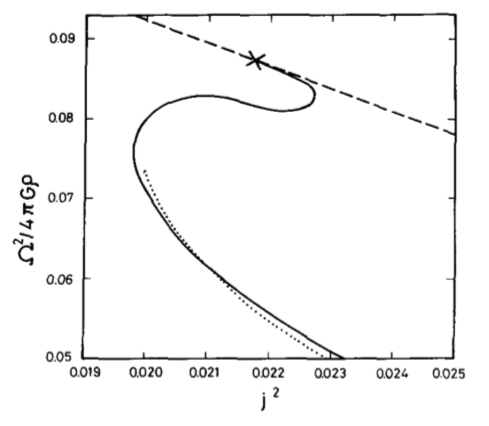CAPTION (modified here):   The squared angular velocity is plotted against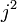$~j^2$ for a segment of the Maclaurin sequence (dashed curve), for the Dyson-Wong sequence (dotted curve), and for the new configurations reported in this 1981 paper by Eriguchi & Sugimoto (solid curve). The "×" mark denotes the neutral point on the Maclaurin sequence against the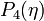$~P_4(\eta)$ perturbation. The dotted curve is plotted by using the values which are read from the curve of Fig. 6 of Wong (1974), so it may contain errors to some extent.

#### Eriguchi & Hachisu (1983)

 Fig. 3 extracted without modification from p. 1134 of Eriguchi & Hachisu (1983) "Two Kinds of Axially Symmetric Equilibrium Sequences of Self-Gravitating and Rotating Incompressible Fluids:Two-Ring Sequence and Core-Ring Sequence" Progress of Theoretical Physics, vol. 69, pp. 1131-1136 © Progress of Theoretical Physics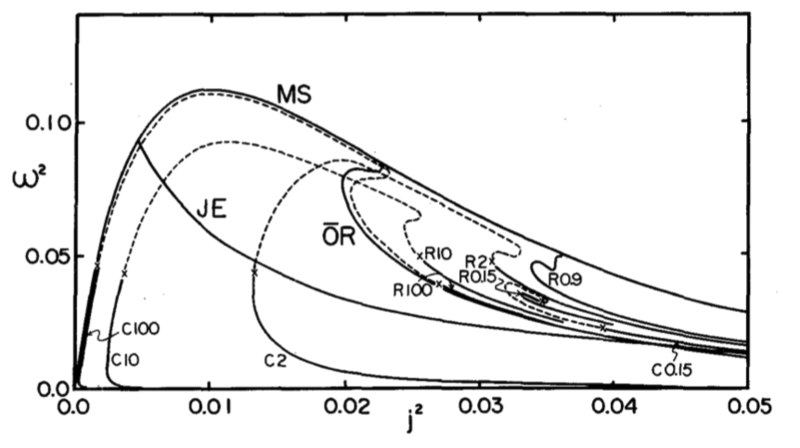CAPTION:  The angular momentum-angular velocity relations. Solid curves represent uniformly rotating equilibrium sequences. MS:   Maclaurin spheroid sequence JE:   Jacobi ellipsoid sequence OR:   one-ring sequence The number and letter R or C attached to a curve denote mass ratio and two-ring or core-ring sequence, respectively. If differential rotation is allowed, the equilibrium sequences may continue to exist as shown by the dashed curves.

#### AKM (2003)

 Fig. 2 extracted without modification from p. 517 of Ansorg, Kleinwächter & Meinel (2003) "Uniformly rotating axisymmetric fluid configurations bifurcating from highly flattened Maclaurin spheroids" MNRAS, vol. 339, pp. 515-523 © Royal Astronomical Society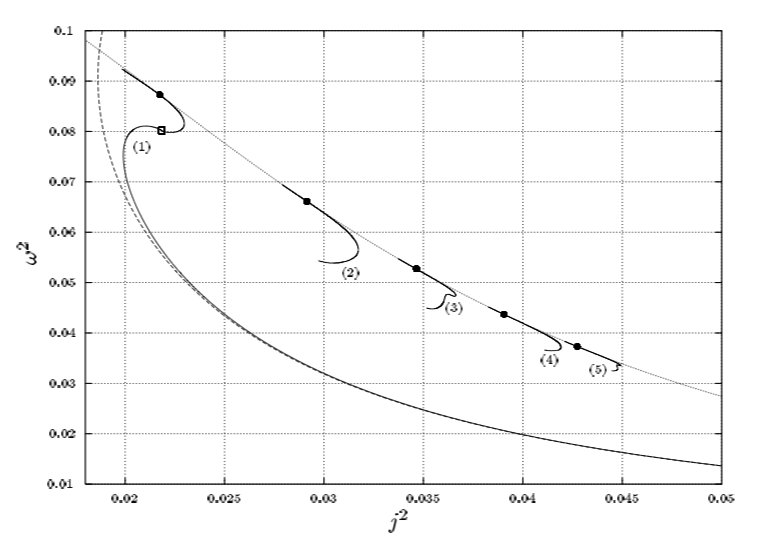CAPTION:   For the first five axisymmetric sequences,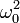$~\omega_0^2$ is plotted against the dimensionless squared angular momentum,$~j^2$, using the same normalizations as Eriguchi & Hachisu (1983). Dotted and dashed curves again refer to the Maclaurin sequence and the Dyson approximation respectively. The full circles mark the bifurcation points on the Maclaurin sequence, and the open square the transition configuration of spheroidal to toroidal bodies on the Dyson ring sequence.

#### Basillais & Huré (2019)

 Fig. 4 extracted without modification from p. 4507 of Basillais & Huré (2019) "Rigidly rotating, incompressible spheroid-ring systems:   new bifurcations, critical rotations, and degenerate states" MNRAS, vol. 487, pp. 4504-4509 © Royal Astronomical Society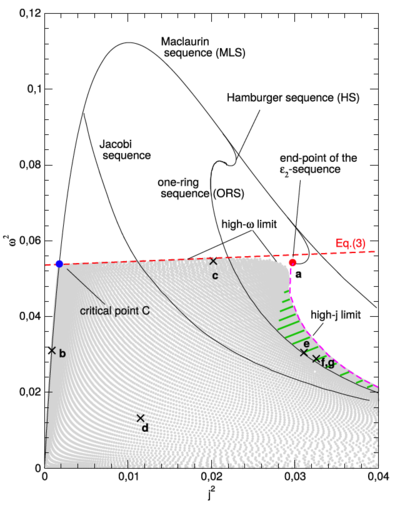CAPTION:   The spheroid-ring solutions (grey dots) populate the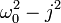$~\omega_0^2 - j^2$ diagram in between the MLS, the high-ω limit, and the high-j limit. The MLS, ORS, Jacobi sequence, Hamburger sequence, and ε2-sequence are also shown (plain lines). Points labelled a to f (cross) correspond to equilibria shown in Figure 3; see also Table 1. There is a band of degeneracy rightward to the ORS (green dashed zone).

##### Central Object

Assume that the central object is exactly a Maclaurin spheroid. Then from Figure 1 (and Table 1) of our review of equilibrium models along the Maclaurin spheroid sequence, we appreciate that all we have to do is specify the eccentricity,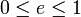$~0 \le e \le 1$, and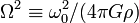$~\Omega^2 \equiv \omega_0^2/(4\pi G\rho)$ is known. For example, if we choose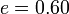$~e = 0.60$, then from that Table 1,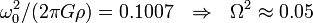$~\omega_0^2/(2\pi G\rho) = 0.1007~~\Rightarrow~~\Omega^2 \approx 0.05$. Other properties of this "central" spheroid — such as its mass, moment of inertia, and angular momentum — are given the the following expressions: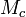$~M_c$$~=$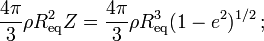$~\frac{4\pi}{3} \rho R_\mathrm{eq}^2 Z = \frac{4\pi}{3} \rho R_\mathrm{eq}^3 (1 - e^2)^{1 / 2} \, ;$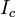$~I_c$$~=$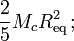$~\frac{2}{5} M_c R_\mathrm{eq}^2 \, ;$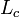$~L_c$$~=$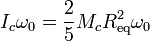$~I_c \omega_0 = \frac{2}{5} M_c R_\mathrm{eq}^2 \omega_0$$~=$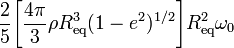$~\frac{2}{5} \biggl[ \frac{4\pi}{3} \rho R_\mathrm{eq}^3 (1 - e^2)^{1 / 2} \biggr] R_\mathrm{eq}^2 \omega_0$$~=$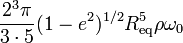$~\frac{2^3\pi}{3\cdot 5} (1 - e^2)^{1 / 2} R_\mathrm{eq}^5 \rho \omega_0$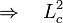$~\Rightarrow ~~~L^2_c$$~=$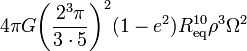$~4\pi G\biggl( \frac{2^3\pi}{3\cdot 5}\biggr)^2 (1 - e^2) R_\mathrm{eq}^{10} \rho^3 \Omega^2$$~=$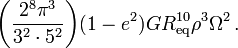$~\biggl( \frac{2^8 \pi^3}{3^2 \cdot 5^2}\biggr) (1 - e^2) G R_\mathrm{eq}^{10} \rho^3 \Omega^2 \, .$

We note as well that the Keplerian frequency for a massless particle orbiting in the equatorial plane of this central object will be,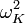$~\omega_K^2$$~=$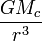$~\frac{GM_c}{r^3}$$~=$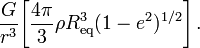$~\frac{G}{r^3} \biggl[ \frac{4\pi}{3} \rho R_\mathrm{eq}^3 (1 - e^2)^{1 / 2} \biggr] \, .$

So, if we force this orbital frequency to also equal the spin-frequency of the Maclaurin spheroid, the radius of the orbit must be,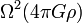$~\Omega^2 (4\pi G\rho)$$~=$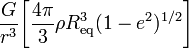$~\frac{G}{r^3} \biggl[ \frac{4\pi}{3} \rho R_\mathrm{eq}^3 (1 - e^2)^{1 / 2} \biggr]$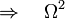$~\Rightarrow~~~\Omega^2$$~=$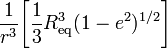$~\frac{1}{r^3} \biggl[ \frac{1}{3} R_\mathrm{eq}^3 (1 - e^2)^{1 / 2} \biggr]$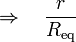$~\Rightarrow~~~\frac{r}{R_\mathrm{eq}}$$~=$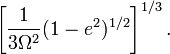$~\biggl[\frac{1}{3\Omega^2} (1 - e^2)^{1 / 2} \biggr]^{1 / 3} \, .$

For example, when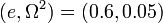$~(e, \Omega^2) = (0.6, 0.05)$, we have,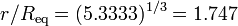$~r/R_\mathrm{eq} = (5.3333)^{1 / 3} = 1.747$.

##### Surrounding Torus

We'll assume that the surrounding 2nd object is a thin torus (1) with the same density as the central object, (2) with a major axis,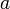$~a$, which ensures that the torus is spinning with the Keplerian frequency prescribed by the mass of the central object, (3) and with a minor cross-sectional radius,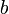$~b$. The second of these constraints means that,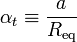$~\alpha_t \equiv \frac{a}{R_\mathrm{eq}}$$~=$$~\biggl[\frac{1}{3\Omega^2} (1 - e^2)^{1 / 2} \biggr]^{1 / 3} \, .$

The mass of the torus is given by the expression,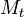$~M_t$$~=$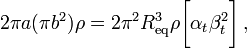$~2\pi a (\pi b^2)\rho = 2\pi^2 R_\mathrm{eq}^3 \rho \biggl[ \alpha_t \beta_t^2 \biggr] \, ,$

where,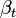$~\beta_t$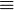$~\equiv$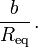$~\frac{b}{R_\mathrm{eq}} \, .$

Given that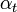$~\alpha_t$ is known once the eccentricity of the central Maclaurin spheroid has been selected and, given that the density of the torus must match the density of the central object, the mass of the torus will only depend on the choice of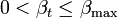$~0 < \beta_t \le \beta_\mathrm{max}$. The maximum allowed value,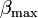$~\beta_\mathrm{max}$, is set by ensuring that equatorial-plane location of the inner edge of the torus is no smaller than the equatorial radius of the central spheroid,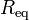$~R_\mathrm{eq}$. This means,$~\beta_\mathrm{max}$$~=$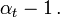$~\alpha_t - 1 \, .$

So, the maximum torus mass is,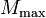$~M_\mathrm{max}$$~=$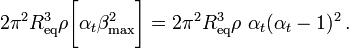$~ 2\pi^2 R_\mathrm{eq}^3 \rho \biggl[ \alpha_t \beta_\mathrm{max}^2 \biggr] = 2\pi^2 R_\mathrm{eq}^3 \rho ~\alpha_t (\alpha_t - 1)^2 \, .$

The moment of inertia of the torus is,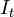$~I_t$$~=$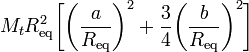$~M_t R_\mathrm{eq}^2\biggl[ \biggl(\frac{a}{R_\mathrm{eq}} \biggr)^2 + \frac{3}{4} \biggl( \frac{b}{R_\mathrm{eq}} \biggr)^2 \biggr]$$~=$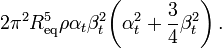$~2\pi^2 R_\mathrm{eq}^5 \rho \alpha_t \beta_t^2 \biggl( \alpha_t^2 + \frac{3}{4} \beta_t^2 \biggr) \, .$

Hence, the (square of the) angular momentum of the torus is,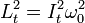$~L_t^2 = I_t^2 \omega_0^2$$~=$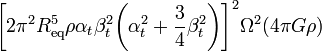$~ \biggl[ 2\pi^2 R_\mathrm{eq}^5 \rho \alpha_t \beta_t^2 \biggl( \alpha_t^2 + \frac{3}{4} \beta_t^2 \biggr) \biggr]^2 \Omega^2 (4\pi G\rho)$$~=$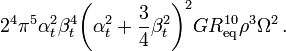$~ 2^4 \pi^5 \alpha_t^2 \beta_t^4 \biggl( \alpha_t^2 + \frac{3}{4} \beta_t^2 \biggr)^2 G R_\mathrm{eq}^{10} \rho^3 \Omega^2 \, .$
##### Combined Configuration

Given that, for the chosen Maclaurin spheroid,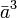$~\bar{a}^3$$~=$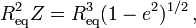$~R_\mathrm{eq}^2 Z = R_\mathrm{eq}^3(1-e^2)^{1 / 2} \, ,$

and that the total mass of the system is,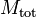$~M_\mathrm{tot}$$~=$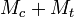$~M_c + M_t$$~=$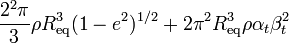$~ \frac{2^2\pi}{3} \rho R_\mathrm{eq}^3 (1 - e^2)^{1 / 2} + 2\pi^2 R_\mathrm{eq}^3 \rho \alpha_t \beta_t^2$$~=$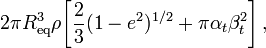$~ 2\pi R_\mathrm{eq}^3 \rho \biggl[ \frac{2}{3} (1 - e^2)^{1 / 2} + \pi \alpha_t \beta_t^2 \biggr] \, ,$

the (square of the) dimensionless total angular momentum of the combined system is,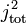$~j_\mathrm{tot}^2$$~\equiv$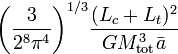$~ \biggl(\frac{3}{2^8 \pi^4}\biggr)^{1 / 3} \frac{(L_c + L_t)^2}{GM_\mathrm{tot}^3 \bar{a}}$$~=$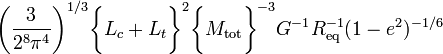$~ \biggl(\frac{3}{2^8 \pi^4}\biggr)^{1 / 3} \biggl\{ L_c + L_t \biggr\}^2 \biggl\{ M_\mathrm{tot} \biggr\}^{-3}G^{-1} R_\mathrm{eq}^{-1} (1 - e^2)^{-1 / 6}$$~=$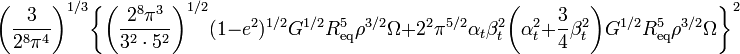$~ \biggl(\frac{3}{2^8 \pi^4}\biggr)^{1 / 3} \biggl\{ \biggl( \frac{2^8 \pi^3}{3^2 \cdot 5^2}\biggr)^{1 / 2} (1 - e^2)^{1 / 2} G^{1 / 2} R_\mathrm{eq}^{5} \rho^{3 / 2} \Omega + 2^2 \pi^{5 / 2} \alpha_t \beta_t^2 \biggl( \alpha_t^2 + \frac{3}{4} \beta_t^2 \biggr) G^{1 / 2} R_\mathrm{eq}^{5} \rho^{3 / 2} \Omega \biggr\}^2$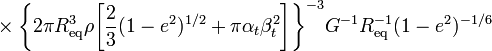$~\times \biggl\{ 2\pi R_\mathrm{eq}^3 \rho \biggl[ \frac{2}{3} (1 - e^2)^{1 / 2} + \pi \alpha_t \beta_t^2 \biggr] \biggr\}^{-3}G^{-1} R_\mathrm{eq}^{-1} (1 - e^2)^{-1 / 6}$

 © 2014 - 2021 by Joel E. Tohline |   H_Book Home   |   YouTube   | Appendices: | Equations | Variables | References | Ramblings | Images | myphys.lsu | ADS | Recommended citation:   Tohline, Joel E. (2021), The Structure, Stability, & Dynamics of Self-Gravitating Fluids, a (MediaWiki-based) Vistrails.org publication, https://www.vistrails.org/index.php/User:Tohline/citation